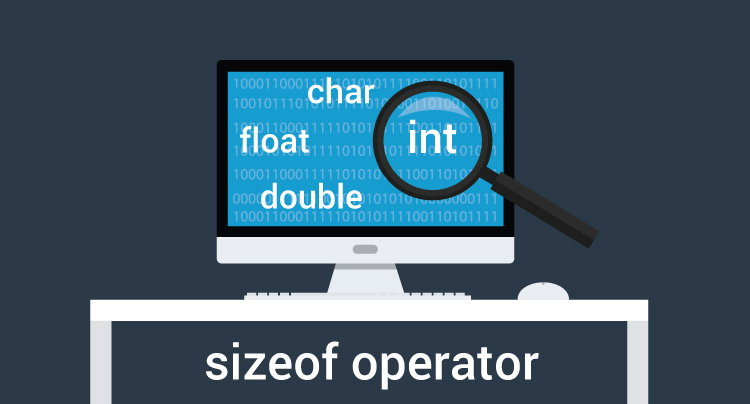C Program to Find the Size of int, float, double and char

This program declares 4 variables of type int, float, double and char. Then, the size of each variable is evaluated using sizeof operator.To understand this example, you should have the knowledge of following C programming topics:

Example: Program to Find the Size of a variable

``````#include <stdio.h>
int main()
{
int integerType;
float floatType;
double doubleType;
char charType;

// Sizeof operator is used to evaluate the size of a variable
printf("Size of int: %ld bytes\n",sizeof(integerType));
printf("Size of float: %ld bytes\n",sizeof(floatType));
printf("Size of double: %ld bytes\n",sizeof(doubleType));
printf("Size of char: %ld byte\n",sizeof(charType));

return 0;
}
``````

Output

```Size of int: 4 bytes
Size of float: 4 bytes
Size of double: 8 bytes
Size of char: 1 byte```

In this program, 4 variables integerType, floatType, doubleType and charType are declared having int, float, double and char type respectively.

Then, the size of each variable is ascertained using sizeof operator.